# Category: Teaching Elementary Math

## Folding Fractions

Supplies Needed:
20 sheets of Blank Paper size: 8 1/2×11
(for approximately 20 students)
Instructions:
1. Break class into 5 groups of 4. Each group has 4 sheets of blank paper.
2. Showing instruction, fold the bottom of the sheet up and to the left creating a right triangle. 3. Cut / tear the top of the folded left over top, removing the top, leaving a perfect square with a fold in it (looking like 2 triangles).
3. Write on each triangle 1/2 and 2/2, denoting the two fractions.
4. With the second sheet of paper, repeat instructions No. 2. This time folding the triangle in the opposite direction. Now there ought to be 4 folds / 4 triangles. Write on each triangle 1/4, 2/4, 3/4, 4/4, denoting the 4 fractions.
5. With the third sheet of paper, repeat instruction No. 2 again. This time folding the paper 3 times. This time there ought to be 8 folds / 8 triangles. Write on each triangle 1/8, 2/8, 3/8, 4/8, 5/8, 6/8, 7/8, 8/8, denoting the 8 fractions.
6. With the fourth sheet of paper, repeat Instruction number 2 again. This time folding the set of triangle 4 times. This time there ought to be 19 folds / 16 triangles. Write on each triangle 1/16, 2/16, 3/16, 4/16, 5/16, 6/16, …etc….up to 16/16, denoting the 16 fractions.
7. Explain that when added each group together, the total equals to the whole number 1.

Question – What is the Lowest Common Denominator?

Check out some of our other Math Games:

http://www.math-lessons.ca/fraction-games-activities/.

## Math and The Chromatic Scale: Loving Music, Loving Math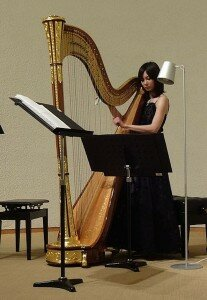Harmony
occurs in music when two pitches vibrate at frequencies in small integer ratios.  Long ago, Greek people realized the concept of harmony occurred when sounds and frequencies are in rational proportion. i.e., One Octave is equal to when the frequency is doubled, and a tripling of frequency brings the key One Octave higher, and is called a perfect fifth. Though not knowing this in relation to “frequency”, ancient Greeks knew this in relation to lengths of vibrating strings; http://www.math.uwaterloo.ca/~mrubinst/tuning/12.html  (Why 12 Notes to The Octave?)

1/1  unison              C

2/1  octave              C

3/2  perfect fifth       G

4/3  fourth              F

5/4  major third         E

8/5  minor 6th           Ab

6/5  minor 3rd           Eb

5/3  major 6th           A

9/8  major 2nd           D

16/9 minor 7th           Bb

15/8  major 7th          B

16/15 minor 2nd          C#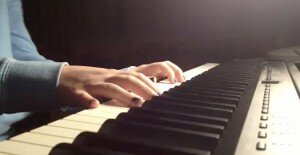The most common conception of the chromatic scale before the 13th century was the Pythagorean chromatic scale. Due to a different tuning technique, the twelve semitones in this scale have two slightly different sizes. (En. Wikipedia. org/wiki/Chromatic_scale)  Thus, the scale is not perfectly symmetric.  http://strathmaths.wordpress.com/2012/02/22/tipping-the-scales-some-of-the-mathematics-behind-music/.  Pythagoras, 13thC Greek mathematician, was famous in geometry for the Pythagorean theorem (en.  Wikipedia. org / wiki/Pythagoras).  The theorem states that in a right-angled triangle, the area of the square on the hypotenuse (the side opposite the right angle) is equal to the sum of the areas of the squares of the other two sides—that is, a^2 + b^2 = c^2.  Pythagoras experimented with a monochord, noticing that subdividing a vibrating string into rational proportions produced resonant sounds. When the frequency of the string is inversely proportional to its length, its other frequencies are simply whole number multiples of the fundamental.  (En. Wikipedia. org/wiki/Chromatic_scale)

The term chromatic derives from the Greek word chroma, meaning color, where the total chromatic / aggregate is the set of all twelve pitch classes; an array being a succession of aggregates.  Shí-èr-lǜ (Chinese: 十二律 (twelve-pitch scale) is a standardized gamut of twelve notes. The Chinese scale uses the same intervals as the Pythagorean scale, based on 2/3 ratios (2:3, 8:9, 16:27, 64:81, etc.). The gamut or its subsets were used for tuning and are preserved in bells and pipes.  In China, the first reference to “the standardization of bells and pitch,” dates back to around 600 BCE.  According to ancient scroll/script literature, Pythagoras taught that music was not intended for entertainment, though for calming the mind and bringing about order from chaos of life and the universe using spiritual instruments.  Music of the Spheres is one of the phrases used to describe Ancient Greek Pythagorean Music.  Here is a sample of what this music sounds like: http://www.youtube.com/watch?v=Bm2Pn_8Oxww  This clip is a short educational video on a Pythagorean Tone Generator: Pythagorean Tone Generator: http://www.youtube.com/watch?v=BhqgOH0gDIc

James Hopkins, a student and practitioner of Pythagorean Monochords visually shows us his handmade Monochord Stringed instruments: http://www.youtube.com/watch?v=tbCZO6rPcY8

For more cool Learning Math Games, feel free to visit us here:

http://www.math-lessons.ca/fraction-games-activities/

http://butterflybooks.ca/math-activities/

## Perimeter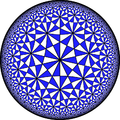The perimeter of a shape is the distance around the sides of the shape. Calculating and distinguishing characteristics of shapes is an important concept in Geometry. Elementary school students should be familiar with both 2-D (square, triangle, rectangle, circle) and 3-D shapes (cube, sphere, cylinder, pyramid, cone). Kindergarteners and first graders should be able to identify the shape by name, whereas 2nd and 3rd graders should be able to calculate the perimeter, and 4th and 5th graders should be well versed in area and volume calculations.

In this activity, students will explore the concept of perimeter and how to calculate the perimeter of regular polygons.

Materials:

Measuring tape

White board and dry erase markers

Note: If your classroom is not perfectly square or rectangular, consider drawing a classroom layout, on the white board that is easy to calculate the perimeter. For more advanced students, challenge them to find the perimeter of their irregularly shaped classroom.

Instructions

1. Have the students measure the dimensions of the classroom (to the nearest foot), drawing a diagram of the classroom.
2. As the students measure the classroom, either have them recreate the classroom on paper, or on the white board in the front of the classroom.
3. Explain to the class that they are calculating the perimeter of the classroom. You may consider saying, “The perimeter are the edges of the classroom that we are measuring. The perimeter tells you the distance around the outside of a shape. The classroom is shaped like a rectangle (or a square), so when do you think it would be helpful to know the distance around the classroom? Yes, if we were going to decorate the classroom with a ribbon, we would need to know the length of the sides of the classroom. The perimeter is an easy calculation to find, just by adding the length of each side together.”
4. Have the students calculate the perimeter of the classroom using their diagrams.

You can extend this activity and encourage the students to measure a room in their home and determine the perimeter of the room. This can also be done measuring the perimeter of the outside playground at the school, or the perimeter of the school’s desks.

For more ideas about how to teach young students about perimeter and distance of shapes, visit: http://www.scholastic.com/teachers/top-teaching/2012/12/10-hands-strategies-teaching-area-and-perimeter and http://www.watchknowlearn.org/Video.aspx?VideoID=32320&CategoryID=3337.

or more fun and interesting Learning Math Games, you can visit us here:
http://www.math-lessons.ca/activities/FractionsBoard5.html
http://www.math-lessons.ca/timestables/times-tables.html
http://www.math-lessons.ca/activities/FractionsBoard4.html

## Probability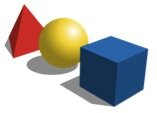What are the chances that you can flip heads or tails? Probability can be a fun guessing game and is an interactive Math concept that can be simple to introduce to elementary school students. Probability can tell you how likely or unlikely an outcome is, which can give you mathematical information to help you make a decision. If you have a 0.00001% chance of finding gold in your backyard, are you going to start digging for gold? (Shapes; downloaded Feb. 7, 2014;  http://commons.wikimedia.org/wiki/File:Basic_shapes.svg)  Probably not, right? Students can begin to learn that outcomes can be numerically calculated and then can convey valuable information.

In this activity students will learn how to calculate and interpret probability.

Materials:

Large bag of assorted color candy

Small plastic bowls

*It is best if you know how many color of each candy is present in the bag. If this is not possible, estimate the approx. numbers of each.

Instructions

1. Assemble the class into small groups.
2. Give each group a small plastic bowl and 1-2 cups of candy in each group’s bowl.
3. Have the students count the total number of candies, the number of candies of each color and record the data on a piece of paper.
4. Explain to the students that the probability of something occurring is another way of saying what are the chances or the likelihood that an event will happen. You may consider saying, “We are going to calculate the probability of you getting a red colored candy from your bowl, if you picked the candy with your eyes closed. The probability is a number that helps you determine if something is likely to happen or not likely to happen. To find the probability of picking a red candy, we need two numbers. The number of red candies in your bowl and the total number of candies in the bowl. In this group, they have 2 red candies and 31 total candies. So the probability would be 2/31, which is a very small number. So, if they were to close their eyes and choose a candy, it would most likely not be red.”
5. Invite students to calculate the probability of picking each color and allow time for them to pick candies at random to explore the concept of probability further.

For older students, extend the activity by practicing how to reduce fractions, for example a probability of 3/18 can be reduced to 1/6. There are lots of fun ways to teach probability. Check out these great online resources:  http://nrich.maths.org/probability and http://www.scholastic.com/browse/article.jsp?id=3756484.

For more fun and interesting Learning Math Games, you can visit us here:
http://www.math-lessons.ca/activities/FractionsBoard5.html
http://www.math-lessons.ca/timestables/times-tables.html
http://www.math-lessons.ca/activities/FractionsBoard4.html

## Writing Mathematical Ratios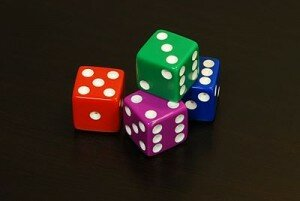Comparing numbers and quantities can be an advanced component of teaching Math, but can also be easily introduced to younger students in first or second grade. In Math, it is important to provide all students with mathematical vocabulary, like ratio or proportion. (Dice; downloaded Feb. 7, 2014; http://en.wikipedia.org/wiki/File:6sided_dice.jpg)  A ratio is nothing more than a comparison of two quantities and we use ratios even outside of the classroom. For example, when cooking, we may use 2 parts flour to 1 part sugar, that would be the ratio 2 : 1 or when mixing cleaning products, like 1/3 cup of bleach to 1 gallon of water. It is essential to explain complex terms like ratio or proportion in easy to understand language, and when possible using hands-on everyday items.

In this activity students will learn how to calculate and write a ratio.

Materials:

5 sealable bags, red/blue/green marbles or unit blocks (20 of each color), white board, dry erase markers *This is best done as a group activity with no more than 4 students per group. Prior to the activity, place a different amount of each marble color in each sealable bag. For example, Bag 1 may have 3 green, 4 blue, and 5 red. Label each bag with a number to better keep track of the bags and marbles used.

Instructions

1. Assemble the class into small groups.
2. Give each group a labeled bag of marbles.
3. Have the students count the total number of marbles in the bag and the number of marbles of each color and record the data on a piece of paper.
4. Have the groups rotate bags so that each group gets a new sealable bag and repeat steps 2 and 3.
5. Ask the students: “What did you notice about the number of marbles in each bag? What about the number of each color?”
6. Explain to the students that the number of each color marble can be written as a ratio or a comparison. You may consider saying, “A ratio is a number that compares to items together. For example, in Bag 1, there were 3 blue marbles and 5 red marbles, so we could compare or write a ratio of blue marbles to red marbles by writing 3 : 5.”

For older students, consider extending the activity by providing them with word problems involving ratios. Have them explore how ratios can be maintained by doubling or halving quantities. For example, you might ask, “if the marbles are to stay in a 3 : 1 ratio, how many marbles of each color would you need if you wanted to double the total number of marbles in Bag 3?”

Ratio activities can be a great tool to teach fractions in the classroom. For more creative math teaching tips, visit: http://nrich.maths.org/4825 and http://mathsnacks.com/teacher.php .

For more fun and interesting Learning Math Games, you can visit us here:
http://www.math-lessons.ca/activities/FractionsBoard5.html
http://www.math-lessons.ca/timestables/times-tables.html
http://www.math-lessons.ca/activities/FractionsBoard4.html
http://www.math-lessons.ca/index.html

## Order of Operations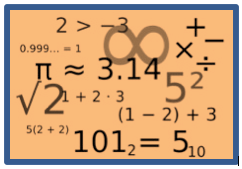Math is like a special type of language that has rules that must be followed. Understanding that the order of numbers and how they are combined is an important concept that elementary school students must master. Just as in everyday activities, like getting dressed, cooking, reading, the order of the steps matter. We would not put our shoes on before putting on socks, or read a book before opening it. Making this concept come to life for young students is easy to do using everyday analogies and hands-on activities.In this activity students will explore the mathematical order of operations.

Materials:

Several clothing items (coat, shirt, socks, hat, sunglasses, gloves)

Whiteboard and dry erase markers

Instructions

1. Invite a volunteer to come to the front of the classroom.
2. Provide them with the various clothing items and explain to the class that even in everyday tasks, like getting dressed, there is a particular order or “rules” that we follow.
3. Ask the student to put the items on (over top of their clothes to simulate getting dressed). As the student does, so point out what item went first, then next.
4. Acknowledge that the order of the hat and sunglasses did not matter. One could either put the sunglasses on first and then the hat or vice versa.
5. Explain how this relates to Math and the order of operations. You may consider saying, “Just like with getting dressed, Math has a special order to it. We do operations that are in parentheses first, then those that may involve exponents, then multiplication and division, then finally, addition and subtraction. However sometimes the order does not matter, like with the sunglasses and hat. When you are only left with addition and subtraction or multiplication and division, you do the operations as they appear from left to right.”
6. Write the following problem on the board: (3 x 5) – 60 ÷ 10 + 7 and walk students through the “order” of what has to be done first, then next, then last. If time permits, write additional problems on the whiteboard and have students work through the order of operations.

Additional Order of Operation Resources: As students graduate to more advanced math, it is essential that they be able to accurately manipulate and calculate values. This is especially true with solving equations or problem-solving. For more interactive ways to introduce and practice the order of operations, visit:

## Teaching Functions and Addends with Fun Machines!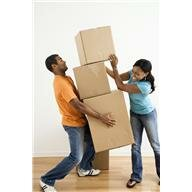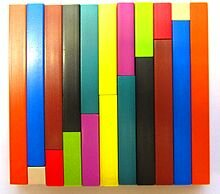This activity may be the most enjoyable way to introduce and practice functions. It can be adapted to meet the needs of a variety of grade levels.

Preparation: You will need a large box (large enough for a student to sit inside of it). Most appliance stores or big box stores will be happy to donate one. You can have the class decorate it (as a recess or free time activity) or you may choose to decorate it yourself. Turn the box upside down and cut out a “doorway” on one side of the box. Then cut two openings (similar to mailbox slots) on the front of the box and identify them as “in” and “out” slots. Once the “Magic Function Machine” is created, it can be used year after year.

Activity:

1)     Have a student sit inside the Magic Function Machine with all needed materials. You can use a variety of items such as counters, dry-erase boards, paper and pencil, craft sticks or any other item you can think of.

2)    The Teacher introduces the rule for the function machine. “This Magic Function Machine is so smart; it always adds 5 to any number you tell it. Who wants to try it out?”

3)    Choose a volunteer to come to the machine and input a number. They can write in on paper, write it on a whiteboard, count out the correct number of counters, etc. They put this number into the “in” slot in the machine and announce to the class what number they gave the machine (“I am putting in 6 craft sticks.”)

4)    Students at their desks figure out what the machine should output while the student in the box does the same.

5)    Give the students time to complete the problem. Then, ask the machine to send the output. “I think we are ready. What is the answer Magic Function Machine?”

6)    The student inside the box sends the answer through the “out” slot. The student outside the box announces the results to the class. (“I put 6 craft sticks in, and the magic machine gave me 11 craft sticks back.”)

Variations:

1)    As students become more proficient, they can create the rule they would like the machine to follow.

2)    Practice missing addends (an important algebraic concept). Have the students figure out the rule rather than the output. (“I put in 7 and 10 came out. What rule is the magic machine using?”)

3)    The complexity of the problems can change based on the abilities of your students. It is simple to individualize this activity.

4)    The problems can vary based on concepts students are practicing (addition, subtraction, multiplication, division, 2-step problems, negative numbers, fractions, etc.)

## Problem Solving Activity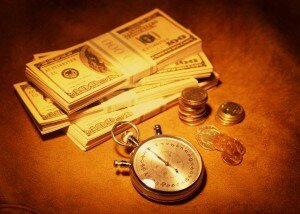Too often, we rely on worksheets to help our students learn. Students need to be active participants in their learning. They need to explore, communicate and problem solve. Here is a fun activity appropriate for second and third graders to complete during your measurement unit. Not only does it help them practice measurement skills (weighing items and counting money), but it also facilitates math process skills such as communication and problem solving.

Materials: scales, envelopes, coins, student directions and recording sheets (attached). Note – you will have to weigh the envelopes you choose to use (the ones I used weighed 3 grams).

Overview: Students work together to determine the amount of money contained in an envelope through problem solving and application of math concepts. If students can successfully determine the amount of money within the envelopes, they are given “credit” to shop for items (erasers, pens, colored pencils, etc.) in their class store.

Class Store

It is your lucky day! Your teacher said she will give you money to shop in the class store. However, there is a catch. She will not tell you how much money you will receive. Instead you need to figure it out. You will be given four different envelopes. Each envelope holds a different type of coin (quarters, dimes, nickels or pennies) which is written on the envelope. No envelopes hold a combination of coins. You must figure out how many coins are in each envelope, how much those coins are worth, and determine how much money you have altogether. Hints are given below.

Fill in the chart completely. If you determine the right amount, your group will be able to spend it in the school store.

Hints:

1 envelope = 3 grams

4 quarters = 23 grams

5 dimes = 11 grams

4 nickels = 20 grams

4 pennies = 10 grams

Complete the chart: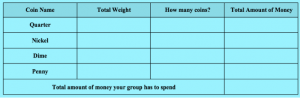Work Space:

For more fun and interesting Learning Math Games, you can visit us here:
http://www.math-lessons.ca/activities/FractionsBoard5.html
http://www.math-lessons.ca/timestables/times-tables.html
http://www.math-lessons.ca/activities/FractionsBoard4.html
http://www.math-lessons.ca/index.html

## Patterning for AlgebraAlgebra used to be viewed as a class for high school students. We know realize the importance of introducing algebraic thinking early in education. Identifying and studying patterns is a significant concept to help the youngest learners develop and enhance algebraic thinking. Creating, completing, identifying and describing patterns help students expand their mathematical mind. Here are some fun patterning activities for your students.

1)    Allow students to create patterns in a variety of ways – Have students create patterns for one another. One student acts out the pattern and chooses a classmate to complete it. If the classmate can correctly continue the pattern, she makes a new one for someone else to continue. Encourage students to be creative using different instruments, movements, etc.

2)    Manipulatives – Pattern Blocks, Attribute Blocks and Color Cubes are all wonderful math manipulatives to use to practice patterning. But don’t limit yourself to these products. Use everyday items such as buttons, crayons, pencils, erasers, stickers and anything else you can imagine.

3)    Problem Solving Activities – One problem solving strategy young students often use involves determining patterns. Here are some examples that can be solved by using patterns.

A)   In a video game, the first score was worth 10 points. The second score was worth 20 points. The third score was worth 30 points. How much was the sixth score worth? Show and describe the pattern that helped you solve this problem.

B)   At the carnival, there was a prize wheel. Each student got to spin one time to see if he or she won a prize. Spinning a “1” won a prize. Spinning a “2” or a “3” did not win a prize. Spinning a “4” won a prize. Spinning a “5” or a “6” did not win a prize. Rita spun a “12.” Did she win a prize? Show and describe the pattern that helped you solve this problem.

C)   Katie’s gym teacher was trying to get them in shape. On the first day, the kids ran 1 lap. On the second day, they ran 3 laps. On the third day, they ran 5 laps. On the fourth day, they ran 7 laps. How many laps did they run on the tenth day? Show and describe the pattern.

D)   The students in the class were lining up for the music concert. The teacher lined them up 1girl, 2 boys, 1 girl, 2 boys. If the teacher continued with this pattern, would the 10th child in line be a boy or a girl? Show and describe the pattern that helped you figure out the problem.

For more fun and interesting Learning Math Games, you can visit us here:
http://www.math-lessons.ca/activities/FractionsBoard5.html
http://www.math-lessons.ca/timestables/times-tables.html
http://www.math-lessons.ca/activities/FractionsBoard4.html
http://www.math-lessons.ca/index.html

## Math Manipulatives in Middle SchoolMost teachers are very comfortable using manipulatives with their youngest students. However, fewer and fewer manipulatives are utilized as students enter middle school, and rote memorization of rules becomes the focus. Yet, Middle School Learners benefit from the use of manipulatives just as much as younger students do. Students find that decimals, fractions and integers are significantly different from the whole numbers they have worked with up to this point. Manipulatives help students explore these new concepts, communicate their thoughts, share examples and truly understand mathematics. Below are three manipulatives every middle school math teacher should be utilizing to help her/his students reach their full potential.  (Pic: Cusinaire Rods in a Staircase Arrangement;  In Wikipedia.  Retrieved March 26, 2013 from http://  en.wikipedia.org/wiki/Mathematical_manipulative

Base-Ten Blocks – Yes, everyone uses base-ten blocks (units , longs  and flats ) with primary students to help them learn place value, counting and operations.

However, base-ten blocks are the perfect tool to teach decimal places as well. Simply reversing the value of each piece (flats ; rods [ ]; units [ ]) allows students to explore smaller numbers in a hands-on fashion.

Fraction Towers– Fraction towers are possibly the best manipulative for middle school students. Fractions are often the most challenged of all middle school math concepts, usually because students do not understand fractions. They are taught to memorize rules, which make no sense to them. Towers allow students to easily compare fractions and complete various operations. Working with fractions in a concrete, visual and hands-on way makes fractions less intimidating for students.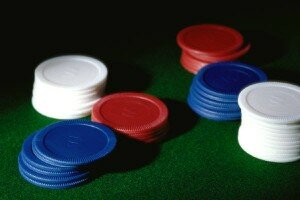Color Chips – Color chips are most often used for statistics and data analysis topics. They are wonderful tools for those topics. However, another great way to use them is with integers. The red side is negative, while the yellow side is positive. You can even take a permanent marker and draw + and – signs on the chips. Students can model adding, subtracting, multiplying and dividing using the chips.

You don’t always need to have the physical materials. Visit the National Library of Virtual Manipulatives at: http://nlvm.usu.edu/en/nav/vlibrary.html to see online versions of these and many other math manipulatives.

And for more of our Fun Learning Math Games, you can visit here:

http://www.math-lessons.ca/activities/index.html

http://www.math-lessons.ca/activities/Geometry.html